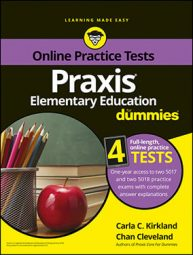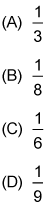##### Praxis Elementary Education For Dummies with Online Practice TestsThe chances are very good that the Praxis Elementary Education exam will contain one or two questions where you'll have to find the probability of an event.

The probability of an event is a ratio that represents the likelihood that it will happen. It is the number of outcomes that qualify as the event divided by the number of possible outcomes. If a bag contains three yellow ping-pong balls, two orange ones, and four green ping-pong balls, the probability of pulling out an orange ping-pong ball when pulling out a ball randomly is 2 out of 9, or

The number of outcomes that could qualify as orange if they happened is 2 because there are two orange balls, and the number of possible outcomes is 9 because there are nine ping-pong balls in the bag.

Now, technically, that is the theoretical probability. It is usually just referred to as "probability," but there is also the principle of experimental probability, which is the number of times an event actually does end up occurring divided by the total number of events. If an orange ping-pong ball were selected 14 out of 15 times, the experimental probability of selecting one would be

The probability that one of two events will happen is the sum of their individual probabilities. The probability that all of two or more events will happen is the product of their individual probabilities.

## Practice question

1. A coin will be flipped three times. What is the probability that it will land on heads all three times?1. The correct answer is Choice (B). The probability that multiple events will all happen is the product of their individual probabilities. Every time a coin is flipped, the probability that it will land on heads is

That is the probability of getting heads for all three coin tosses.

Choice (A) is 1 over the number of coin tosses. Choice (C) is 1 over the sum of the denominators of the individual probabilities. Choice (D) is 1 over the square of the number of coin tosses.